Next: Empirical results Up: Short Term Prediction of Previous: Sale forecast by

# Preprocessing the input data

An efficient preprocessing of the data is necessary to input it into the net. Due to our implementation of the back-propagation algorithm all information must be scaled to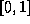. We assume that the necessary information is given for T weeks in the past. With the following definitionswe have decided to use the following inputs for each article i and week t:For each article i and recent week t we use the three-dimensional vector: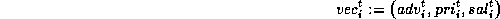For a week t in the future the vector is reduced by the unknown sale: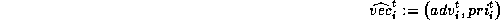To predict the sale for one article within a week t, we use a window of the last n weeks. So we have the following input vector for each article i: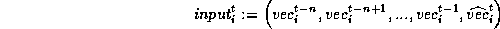We have quite a constant sales volume of all products, because all the considered articles belong to one product group. An increasing sale of one article in general leads to a decrease of other sales. Due to this, we concatenate the input vectors of all p articles to get the vector given to the input layer: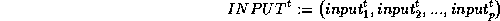The sale of article i within week t (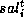) is the requested nominal value in the output layer that has to be learned by one net for this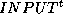vector. So we have p nets where the i-th net adapts the sale behaviour of article i. Therefore we have a training set with the following pairs (see figure 1):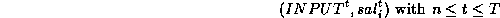To forecast the unknown sale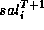for any article i within a future week T+1 we give the following input vector to the trained i-th net: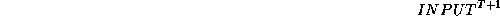The output value of this net is expected to be the value, which has to be re-scaled to the value for the sale of article i within week T+1: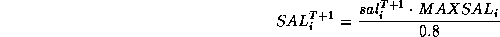Next: Empirical results Up: Short Term Prediction of Previous: Sale forecast by